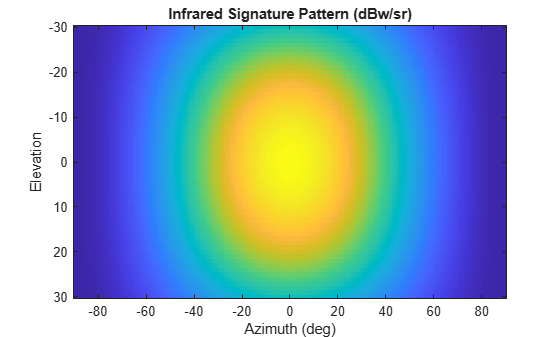# value

Infrared intensity at specified angle and frequency

## Syntax

``irval = value(irsig,az,el)``

## Description

example

``` `irval = value(irsig,az,el)` returns the value of the IR intensity, `irval`, specified by the IR signature object, `irsig`, computed at the azimuth, `az`, and elevation, `el`. If the specified azimuth and elevation is outside of the region in which the IR signature is defined, the IR intensity is returned as `-Inf` in dBw/sr.```

## Input Arguments

expand all

Radar cross-section signature, specified as an `irSignature` object.

Azimuth angle, specified as scalar or length-M real-valued vector. Units are in degrees. The `az`, `el`, and `freq` arguments must have the same size. You can, however, specify one or two arguments as scalars, in which case, the arguments are expanded to length-M.

Example: `30`

Data Types: `double`

Elevation angle, specified as scalar or real-valued length-M vector. The `az` and `el` arguments must have the same size. You can, however, specify one or two arguments as scalars, in which case, the arguments are expanded to length-M. Units are in degrees.

Example: `-4`

Data Types: `double`

## Output Arguments

expand all

Infrared intensity, returned as a scalar or real-valued length-M vector. Units are in dBw/sr.

## Examples

expand all

Create and display an IR intensity signature. The signature depends on azimuth and elevation.

Define the azimuth and elevation angle sample points.

```az = -90:90; el = [-30:30];```

Create the IR intensity signature pattern.

```pat = 50*cosd(2*el.')*cosd(az).^2; irsig = irSignature('Pattern',pat,'Azimuth',az,'Elevation',el);```

Display the IR pattern.

```imagesc(irsig.Azimuth,irsig.Elevation,irsig.Pattern) xlabel('Azimuth (deg)') ylabel('Elevation') title('Infrared Signature Pattern (dBw/sr)')```Get the IR intensity value at 25 degrees azimuth and 10 degrees elevation.

`value(irsig,25,10)`
```ans = 38.5929 ```

Get IR intensity value outside of the valid elevation span.

`value(irsig,25,35)`
```ans = -Inf ```

## Version History

Introduced in R2018b